# Autoencoder: Downsampling and Upsampling

Friday, February 15, 2019

Note: Read the post on Autoencoder written by me at OpenGenus as a part of GSSoC.

An autoencoder is a neural network that learns data representations in an unsupervised manner. Its structure consists of Encoder, which learn the compact representation of input data, and Decoder, which decompresses it to reconstruct the input data. A similar concept is used in generative models.

Given a set of unlabeled training examples $${x_1,x_2,x_3,…}$$, an autoencoder neural network is an unsupervised learning algorithm that applies backpropagation, setting the target values to be equal to the inputs. i.e., it uses $$y_i=x_i$$.

For example, in case of MNIST dataset,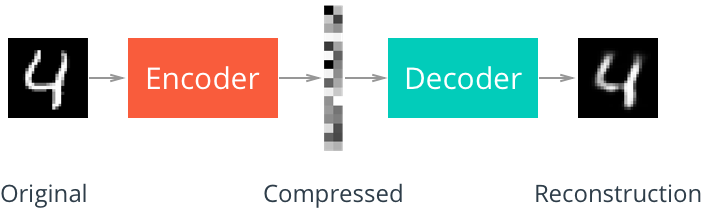## Linear autoencoder

The Linear autoencoder consists of only linear layers.

In PyTorch, a simple autoencoder containing only one layer in both encoder and decoder look like this:

## Convolutional autoencoder

In Convolutional autoencoder, the Encoder consists of convolutional layers and pooling layers, which downsamples the input image. The Decoder upsamples the image. The structure of convolutional autoencoder looks like this: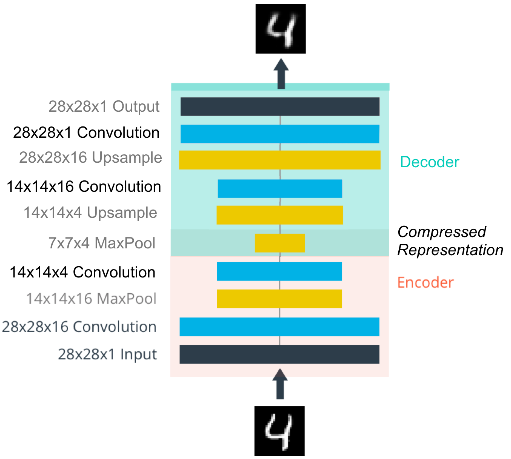Let’s review some important operations.

### Downsampling

The normal convolution (without stride) operation gives the same size output image as input image e.g. 3x3 kernel (filter) convolution on 4x4 input image with stride 1 and padding 1 gives the same-size output.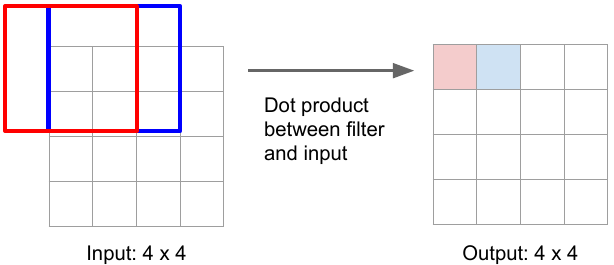But strided convolution results in downsampling i.e. reduction in size of input image e.g. 3x3 convolution with stride 2 and padding 1 convert image of size 4x4 to 2x2.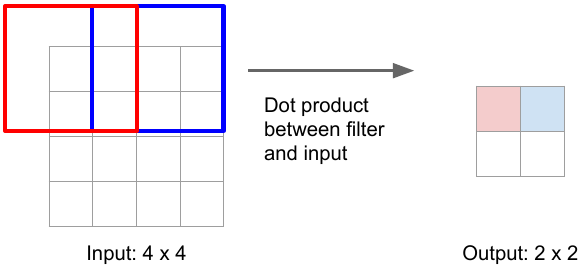### Upsampling

One of the ways to upsample the compressed image is by Unpooling (the reverse of pooling) using Nearest Neighbor or by max unpooling.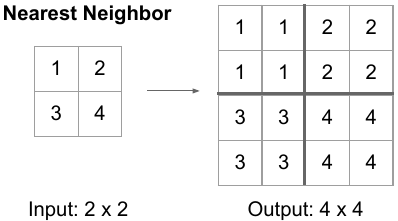Another way is to use transpose convolution. The convolution operation with strides results in downsampling. The transpose convolution is reverse of the convolution operation. Here, the kernel is placed over the input image pixels. The pixel values are multiplied successively by the kernel weights to produce the upsampled image. In case of overlapping, the values are summed. The kernel weights in upsampling are learned the same way as in convolutional operation that’s why it’s also called learnable upsampling.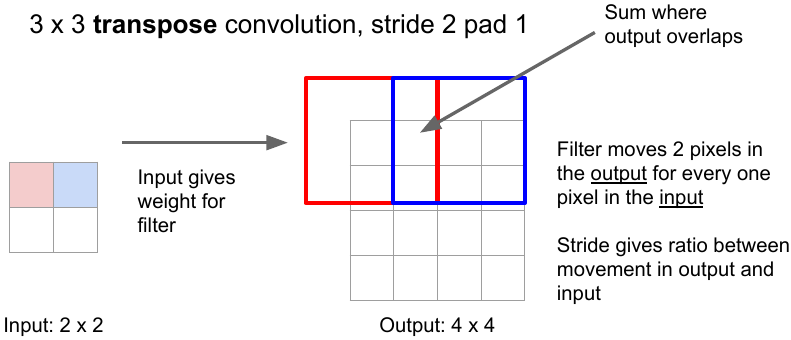One other way is to use nearest-neighbor upsampling and convolutional layers in Decoder instead of transpose convolutional layers. This method prevents checkerboard artifacts in the images, caused by transpose convolution.

## Denoising autoencoders

The denoising autoencoder recovers de-noised images from the noised input images. It utilizes the fact that the higher-level feature representations of image are relatively stable and robust to the corruption of the input. During training, the goal is to reduce the regression loss between pixels of original un-noised images and that of de-noised images produced by the autoencoder.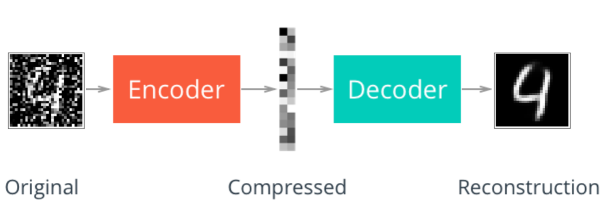There are many other types of autoencoders such as Variational autoencoder (VAE).

References: